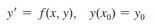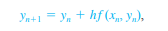Need Help?

Subscribe to Differential Equation

###### \${selected_topic_name}
• Notes
• Comments & Questions
• الـ (numerical method) طريقة عددية تُستخدم في حل الـ first order DE
• الطريقة الأولى باستخدام الـ tangent line وهتكون الـ First order initial value problem على الشكلبحيث الـ equation of tangent line على الشكل y = L (x)

• الطريقة الثانية هي Euler's Methodوالـ  general form بيكون على الشكلNo comments yet